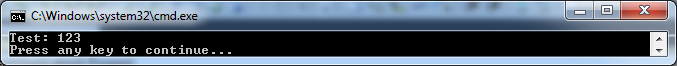# Calling a non-dynamic method with parameters from a dynamic method

## Posted by Filip Ekberg on 14 Oct 2011

I think it's time that we explore some more `OpCodes` and in this post I will look at how we can call methods with parameters passed to them!

There are two `OpCodes` that we can use to execute a method these are:

The difference between these two are important, the first one `OpCodes.Call`, calls a method and expects that we are coming back after the `OpCodes.Ret` in the called method. Whereas `OpCodes.Jmp` exit the context.

Also the Jmp operation has a set of requirements ( from MSDN ):

• There are no stack transition behaviors for this instruction.
• The jmp (jump) instruction transfers control to the method specified by method, which is a metadata token for a method reference. The current arguments are transferred to the destination method.
• The evaluation stack must be empty when this instruction is executed. The calling convention, number and type of arguments at the destination address must match that of the current method.
• The OpCodes.Jmp instruction cannot be used to transferred control out of a try, filter, catch, or finally block.

So by the looks of it, we want to use `OpCodes.Call`! The method that I want to call is quite simple, it looks like this:

``````public static void Test(int a)
{
Console.WriteLine("Test: {0}", a);
}
``````

The method takes a parameter and prints `"Test: {The Value of a goes here}"`, so if we would call `Test(10)` we would get this printed in the console:

``````Test: 10
``````

We've seen how we can read arguments with operation codes, but how do we actually emit and operation code with a certain value, the value that we want to pass to the method? There's an operation code called `Ldc_I4` and it basically means the following:

Pushes a supplied value of type int32 onto the evaluation stack as an int32.

This is exactly what we are looking for! So we got the operation that we want to emit in order to solve the argument issue, but how do we actually call our method? It turns out that the operation `OpCodes.Call` expects a parameter passed to the emit method and in this case it's expecting information about the method that we want to call. And how do we pass information about the method?

By using MethodInfo of course!

Let's first of all compose the `DynamicMethod` before we start emitting IL, it will look somewhat like this:

``````var someMethod = new DynamicMethod(
"SomeMethod",
typeof(void),
null,
typeof(Program).Module);
``````

The third variable is `null` because I don't want any parameters sent to my dynamic method at the time being! Okay so we need to do two more things before we actually start emitting the IL, this includes getting the actual IL generator and all methods in our current class:

``````ILGenerator il = someMethod.GetILGenerator();
var methodToCall = typeof(Program).GetMethods();
``````

Now let's start emitting IL!

The first thing that I want to emit is the argument that I will be passing to the method and this will look like this:

``````il.Emit(OpCodes.Ldc_I4, 123);
``````

So I am saying that I want to push a 32bit integer and the value of the integer is 123. Next up we'll be doing the actual call and for this I need to get the method information from the above methods collection and then I will just return from the method:

``````il.Emit(OpCodes.Call, methodToCall.First(x => x.Name == "Test"));
il.Emit(OpCodes.Ret);
``````

Now everything is set, we have our dynamic method ready and all we need to do know is to create the delegate, but we don't have a deleaget that we've defined? No worries! We can use `Action`:

``````var toInvoke = (Action)someMethod.CreateDelegate(typeof(Action));
``````

And now we can call this just like we normally do:

``````toInvoke();
``````

The result will look like this: